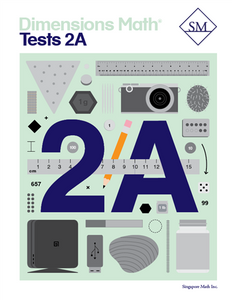# Dimensions Math Tests 2A

Vendor
Singapore Math Inc.
Regular price
\$13.70
Sale price
\$13.70
Quantity must be 1 or more

The Dimensions Math® PK-5 series is a new curriculum designed by Singapore Math Inc. to better serve U.S. teachers and students. Aspects of Singapore math curriculum have been updated for clarity and relevance, while preserving the solid foundation that makes it unique. The series follows the principles outlined in the Singapore Mathematics Framework and uses the Concrete > Pictorial > Abstract approach. The curriculum also incorporates Singapore mental math strategies and model drawing.

In the Dimensions Math program, concepts are introduced and explained in a new format with vibrant imagery. The implementation of this curriculum suits today’s needs, while the progression and scope that define Singapore math remain intact.

The Dimensions Math Tests books offer a series of assessment to help teachers and parents systematically evaluate student progress.

Samples of Dimensions Math Tests 2A

Chapter 1: Numbers to 1,000
Test A
Test B

Chapter 2: Addition and Subtraction — Part 1Test A
Test B

Chapter 3: Addition and Subtraction — Part 2
Test A
Test B

Chapter 4: Length
Test A
Test B

Chapter 5: Weight
Test A
Test B

Continual Assessment 1
Test A
Test B

Chapter 6: Multiplication and Division

Test A
Test B

Chapter 7: Multiplication and Division of 2, 5, and 10

Test A
Test B

Continual Assessment 2
Test A
Test B